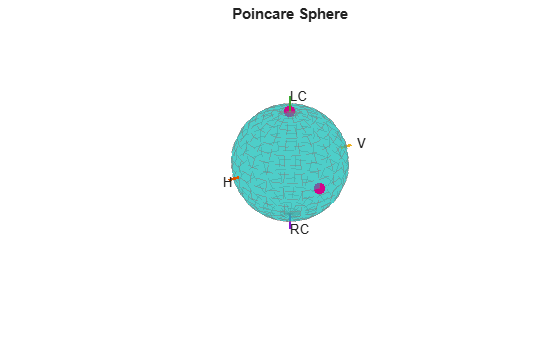Main Content

# stokes

Stokes parameters of polarized field

## Syntax

``G = stokes(fv)``
``stokes(fv)``

## Description

example

````G = stokes(fv)` returns the four Stokes parameters `G` of a polarized field or set of fields specified in `fv`. The field should be expressed in terms of linear polarization components. The expression of a field in terms of a two-row vector of linear polarization components is called the Jones vector formalism.```

example

````stokes(fv)` displays the Stokes parameters corresponding to `fv` as points on the Poincare sphere.```

## Examples

collapse all

Create a left circularly-polarized field. Convert it to a linear representation and compute the Stokes vector.

```cfv = [2;0]; fv = circpol2pol(cfv); G = stokes(fv)```
```G = 4×1 4.0000 0 0 4.0000 ```

Display points on the Poincare sphere for a left circularly-polarized field and a 45° linear polarized field.

```fv = [sqrt(2)/2, 1; sqrt(2)/2*1i, 1]; G = stokes(fv)```
```G = 4×2 1.0000 2.0000 0 0 0 2.0000 1.0000 0 ```
`stokes(fv);`The point at the north pole represents the left circularly-polarized field. The point on the equator represents the 45° linear polarized field.

## Input Arguments

collapse all

Field vector in its linear polarization representation specified as a 2-by-N complex-valued matrix or in its linear polarization ratio representation specified as a 1-by-N complex-valued row vector. If `fv` is a matrix, each column of `fv` represents a field in the form `[Eh;Ev]`, where `Eh` and `Ev` are its horizontal and vertical linear polarization components. The expression of a field in terms of a two-row vector of linear polarization components is called the Jones vector formalism. If `fv` is a vector, each entry in `fv` is contains the polarization ratio, `Ev/Eh`.

Example: [sqrt(2)/2*1i; 1]

Data Types: `double`
Complex Number Support: Yes

## Output Arguments

collapse all

`G` contains the four Stokes parameters for each polarized field specified in `fv`. The Stokes parameters are computed from combinations of intensities of the field:

• G0 describes the total intensity of the field.

• G1 describes the preponderance of horizontal linear polarization intensity over vertical linear polarization intensity.

• G2 describes the preponderance of +45° linear polarization intensity over -45° linear polarization intensity.

• G3 describes the preponderance of right circular polarization intensity over left circular polarization intensity.

 Mott, H., Antennas for Radar and Communications, John Wiley & Sons, 1992.

 Jackson, J.D. , Classical Electrodynamics, 3rd Edition, John Wiley & Sons, 1998, pp. 299–302.

 Born, M. and E. Wolf, Principles of Optics, 7th Edition, Cambridge: Cambridge University Press, 1999, pp 25–32.

## Support

#### Exploring Hybrid Beamforming Architectures for 5G Systems

Download white paper